# Integration by parts twice

By Murray Bourne, 11 Nov 2010

When we first learn how to integrate, the examples we see involve simple polynomials, or single functions like these: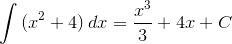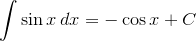## Integrals of products

What if we need to find the integral of a product of 2 functions, like the following example?

Example 1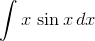This is where we need the important and useful technique in calculus known as integration by parts. (You can see a full explanation starting from basic principles and with more examples here: Integration by parts).

To find this integral, we choose "u" such that its derivative is simpler than u. In this case, we will choose u = x and proceed as follows:

 u = x dv = sin x dx du = dx v = −cos x

We apply the integration by parts formula and find the integral: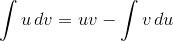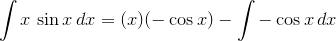Tidying this up gives: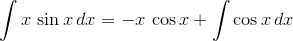Now, that last integral is easy and we can write our final answer: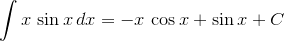Note 1: The constant of integration (C) appears after we do the final integration.

Note 2: Choosing u and dv can cause some stress, but if you follow the LIATE rule, it is easier. For u, choose whatever comes highest in the folloentrwing list, and choose dv as the lowest in this list.

L - logarithm functions
I - Inverse trigonometric functions
A - Algebraic functions (simple polynomial terms)
T - trigonometric functions
E - Exponential functions

## Integration by parts - twice

Now, let's see a case that is double-barreled. That is, we don't get the answer with one round of integration by parts, rather we need to perform integration by parts two times.

Example 2: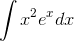In this example we choose u = x2, since this will reduce to a simpler expression on differentiation (and it is higher on the LIATE list), where ex will not.

 u = x2 dv = exdx du = 2x dx v = ex

Now for integration by parts: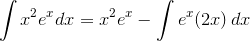We re-arrange this to give the following, which I call equation :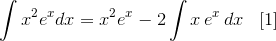This time we can't immediately do that final integral, so we need to perform integration by parts again. Choosing "u" so that its derivative is simpler than u again, we have:

 u = x dv = ex dx du = dx v = ex

Note that the u and v here have different values from the u and v at the beginning of Example 2. This can be a trap if you don't write things carefully!

Now we proceed using integration by parts on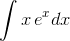: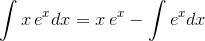That last integral is simple, and we get the following, which I call equation :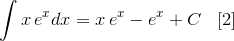But we haven't finished the question - we must remember we are finding this integral:This was our answer to the first integration by parts:Substituting answer  this into equation gives us: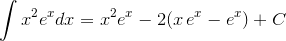Tidying this up, we obtain the final answer: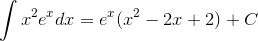Notice the place where the constant "+C" appears in our answer - it's after thel integration has been performed. (Some students get hung up on this step, or add the "+C" before it is appropriate, and some forget to add it at all!) I have used a subscript 1 on the first constant since it is not the same value as the final C.

## Integration by parts twice - with solving

We also come across integration by parts where we actually have to solve for the integral we are finding. Here's an example.

Example 3: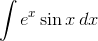In this example, it is not so clear what we should choose for "u", since differentiating ex does not give us a simpler expression, and neither does differentiating sin x. We choose the "simplest" possiblity, as follows (even though ex is below trigonometric functions in the LIATE table):

 u = ex dv = sin x dx du = ex dx v = −cos x

Apply the integration by parts formula:We obtain the following, which I'll call equation :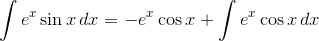Now, for that final integral: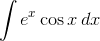Once again, we need to decide which function to use for u, and settle on the one which gives simplest derivative:

 u = ex dv = cos x dx du = ex dx v = sin x

Applying integration by parts for the second time:We obtain equation :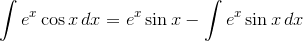Wait a minute - we have a final integral that is the same as what we started with! If we kept going, we would go around in circles and never finish.

So we need to perform the following "trick". We substitute our answer for the second integration by parts (equation ) into our first integration by parts answer (equation .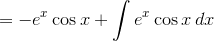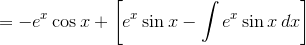Removing the brackets: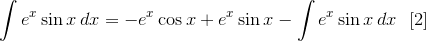Now, this equation is in the following form:

p = −q + rp

To solve this for p, we just add p to both sides:

2p = −q + r

Then divide both sides by 2:

p = (−q + r)/2

So we will do the same to our integral equation, number .

I addto both sides: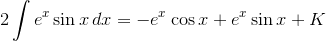Dividing both sides by 2 gives: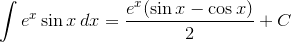So we have solved equation  for, giving us the desired result.

(Note I used a "+K" for the first constant that appeared. My final "C" has value K/2, but normally we only need to be concerned with the final constant.)

### 31 Comments on “Integration by parts twice”

1. Pseudonym says:

I've done a lot of integrals by hand in my time, but I don't think I've used integration by parts even once the last 15 years. Let me explain why.

Integration by parts is essentially the product rule for differentiation inverted:

d(uv)/dx = du/dx v + u dv/dx
⇔ ∫ d(uv)/dx dx = ∫ du/dx v dx + ∫ u dv/dx dx
⇔ uv = ∫ du/dx v dx + ∫ u dv/dx dx
⇔ ∫ du/dx v dx = uv - ∫ u dv/dx dx

The problem with applying integration by parts is that it's often not obvious what to choose for u and v, and even when it is, there are usually constant factors and exact forms which are tricky to get "right".

Instead, I use the Risch method: Determine a generic product form for the integrand, then differentiate.

Let me explain what I mean.

Consider:

∫ (x² + 3x + 21)e^x dx

What we have is a polynomial in x multiplied by e^x. Now what would happen if we differentiated that?

d/dx [p(x) e^x]
= p'(x) e^x + p(x) e^x
= (p'(x) + p(x)) e^x

Now isn't that interesting? If p(x) has degree k, then the derivative of p(x) e^x is another polynomial, also of degree k, multiplied by e^x.

So this should also be true if we integrate! That is, there should be some polynomial p(x) such that:

∫ (x² + 3x + 21)e^x dx = p(x) e^x + C

So let's let p(x) be a generic quadratic polynomial:

p(x) = a2 x² + a1 x + a0

Then:

∫ (x² + 3x + 21)e^x dx = (a2 x² + a1 x + a0) e^x + C

Differentiate both sides, noting that dC/dx = 0.

(x² + 3x + 21)e^x = (a2 x² + a1 x + a0) e^x + (2a2 x + a1) e^x
⇔ x² + 3x + 21 = a2 x² + (a1 + 2a2) x + (a0 + a1)

Matching up coefficients of x gives:

a2 = 1
a1 + 2a2 = 3
a0 + a1 = 21

This is a system of linear equations, three equations in three unknowns. The solution is:

a2 = 1
a1 = 1
a0 = 20

That is:

∫ (x² + 3x + 21)e^x dx = (x² + x + 20) e^x + C

And that's it. We didn't have to "do" integration by parts once.

Of course, we could now fake it if we wanted to. In integration, knowing the answer is most of the battle; once you have the right answer, you insert whatever valid reasoning you want to justify it. So if the question asked for integration by parts, you could do that now, and you'd be more likely to get the right answer.

The difficult bit about this method is recognising what "most general form" for the integrand is appropriate. There is a more-or-less systematic way to do this, and most computer algebra systems implement exactly this method. But it requires a little bit of knowledge sometimes.

For example, this integral:

∫ e^x sin x dx

requires noticing that for some constants α, β, γ and δ:

d/dx [e^x (α sin x + β cos x)]
= e^x (γ sin x + δ cos x)

That is, you have to include sin and cos, even though cos doesn't appear in the integral that you have to perform. But the good news is that the method detects its own errors. If your form wasn't general enough, you'll just get a system of equations with no solutions.

2. Murray says:

Thanks for the method, Pseudonym! There is certainly more than one way to skin a cat. Some readers will balk at the simultaneous equations step in the middle, but it is not difficult.

More fundamentally, though, I suspect less and less people are doing any integrations by hand. There are so many good tools out there now, including Wolfram|Alpha.

Is integration by hand going the way of the dinosaur?

3. Dalcde says:

Anyone doing calculus should not have problems with simultaneous equation.

4. Murray says:

@Dalcde: You are right with the word "should", but the reality is usually something else...

5. sam says:

6. april says:

@murray: yes, u are right. there are lots of devices that do integrations..and actually my calculator has a integration. so, i do use this to check if my calculations are right..
but in our calculus, we always do integration at hand and such devices are just use for checking...

@Dalcde: murray is right.. we do study calculus, but we still have a hard time of doing integrations especially the complex ones.

7. Mridul Gupta says:

I do not agree with you guys at all

Earlier i depended on wolfram for checking/confirming my indefinite integration problems....but since that time i have realized that it just provides integration of very simple functions. Let me give you guys a good example which wolfram cant even calculate in its dreams, but you guys can do it by hand very easily

it is integration of 1 / [sin^5(x)+ cos^5(x)]

I can give many such examples(or you can take it as good questions for practice) which wolfram can never calculate.

Speaking truth, i love doing indefinite integration because it requires an attempt from my side and not just a machine

No grudges against anyone, just putting forward my view. I apologize if it hurts someone.

8. Murray says:

No one is hurt! Certainly there are some integrals that are better done by hand than by machine, since the latter can give us the answer in a pretty messy form.

But I'm not sure why you say Wolfram|Alpha cannot do the integral you mentioned. Here is its solution.

9. Pseudonym says:

I think Mridal Gupta's point is that Mathematica's solution is a lot less helpful than any answer you'd get by hand.

But as a general comment: If you want to check your answer, don't ask Mathematica or Alpha, just differentiate! Differentiation is easy.

Incidentally, I agree with Dalcde. A simple linear system like this should not be difficult for someone doing integral calculus. If it is, then this is a bad comment on the education system.

But then, I never learned trig identities before doing calculus, either. I've still only memorised two of them. If I need any others, I derive them.

10. Mridul Gupta says:

Yeah, the complexities of the solution given by wolfram can scare anyone (at least i got scared when I saw that)

and pardon Pseudonym its 'Mridul' not 'Mridal' 🙂

11. Thank you says:

wow!!! this helps me out A LOT!!! thank you very much!!!! i'm so happy now 😀

12. nasiriyan says:

thank you so much
this helps me out a lot.

13. JD says:

In simplifying from this step:

\int{x^2e^x}dx={x^2}e^x-2(x\,e^x-e^x)+C

It seems like you forgot to foil in the -2 to everything within the parenthesis, because you report your next answer as

\int{x^2e^x}dx=e^x(x^2-2x-1)+C

In actuality the last part should be = e^x(x^2-2x+2) +C

14. Murray says:

@JD - Thanks for pointing that out. It's fixed now.

15. Math Tutor says:

I don't believe (ln x)(cos x) can be integrated using integration by parts or u substitution or some combination thereof.

Is there a general rule/principal regarding when the product of two elementary functions can be integrated? For example a polynomial with positive exponents times a trig or exponential will always be integrate-able b/c the polynomial can derive to zero and the other function (ie e^x or a trig function) can be integrated repeatedly so either integration by parts or tabular integration can be used (I guess those are actually the same come to think of it). But ln x is different b/c it cannot be derived to zero.

16. Murray says:

@Math Tutor: You are right - your example cannot be integrated via integration by parts.

I guess the "general rule" is almost what you have already stated - that is, we need to be able to differentiate one of the parts of the integrand so it eventually disappears (or reappears, as in the case of some trigonometric functions, so they can be re-substituted) and be able to integrate the other part.

You may find this paper interesting:
A counterexample to integration by parts

17. michelle chikomo says:

felt like crying last night when i attempted the integration by parts homework exercise given to us at uni, then i came across this website. THANK YOU SO MUCH. took 20 minutes for me to understand this whole section after going through these notes #HAPPYUNISTUDENT

18. Murray says:

@Michelle: You are very welcome! I'm glad it helped.

19. John Roberto says:

Isn't it supposed to be ∫u dv=uv−∫v du + C because you got the antiderivative of d(uv)? Why is it that you only include the constant after integrating the "v du" part of IBP?

20. Murray says:

@John: It's a good question! We normally put the "+C" after we have done all the integrations involved in a problem.

Otherwise, we would need to put something like +C1 for the place you mentioned, +C2 for the left hand integral and then +C3 for the right hand one. But in this work, it's just some arbitrary constant.

Hope it makes sense.

21. John Roberto says:

Thank you for clearing it up for me! (Sorry for the late reply)

22. Mahesh says:

While it is possible to add an integral on both sides, Constants have to be taken care of. Integrating dx/x by taking u = 1/x and dv = dx
gives du = -1/x^2 and v = x.
$u dv = uv -$ v du
$dx/x = 1 -$ x*(-1/x^2)
Ln x = 1 + Ln x

23. Murray says:

@Mahesh: As mentioned in the article, we add the constant of integration after the last integration step (it is "subsumed" in the solution up to that point.)

Your last line is not really possible for any value of x.

24. ALESSIO says:

Hi there,
I am studying Integration by Parts and I do not understand how to recognise when a Function must be integrated twice or just once. In my book says that when is still a product must be re-integrated again, but in an exercise I had COSXe^3x-xCOSe^3x and between COSX and e^3x it was a product but it didn't re-integrate. So my issue is there is a way on recognising when to integrate twice and when not?

25. Murray says:

@Alessio: Actually, this would be a good question for the IntMath Forum.

Essentially, if your answer still contains an integral sign, then you need to integrate once more (or possibly more than once). The whole idea of integration by parts (like a lot of the integration methods you use) is to break down harder problems into easier ones.

Once we have found all the possible integrals, we can quit.

Does your question mean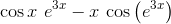?

26. mhlengi says:

How would you do this one though... cosxe^3x - xcos(e^3x)
it got me stuck!!

27. Murray says:

@mhlengi: Your question appears to be identical to Alessio's, so the same advice follows!

28. waseem says:

sir we have to need to intigrate by parts .please tell me the reason behind this . and why we intigrate in by parts.

29. Murray says:

@Waseem: As suggested by the opening sentence of the Integration by Parts lesson, we need it for cases where we are integrating the product of two functions. For many such cases, integration by parts is the only way we can break it down into something simpler (which is the point of most of the integration techniques we learn).

30. precious says:

pls how do we recongnise integral by sustitution,integral by part,normal integrate

31. Murray says:

@precious: (1) As soon as you see one of the square root expressions in the box at the top of the Trigonometric Substitution page, then try to do it by trig substitution.

(2) If you see a product of functions (something like x sin x), then it's likely to be integration by parts.

(3) Finally, "ordinary" integration will involve "simple" functions only (no powers of functions).

My best advice is to keep an open mind. Try it using the method that is suggested by the above summary, but if it doesn't seem to be working, abandon it for a while and try the next most likely.

Over time, as you do more of them, you can usually spot which type it is more confidently.

### Comment Preview

HTML: You can use simple tags like <b>, <a href="...">, etc.

To enter math, you can can either:

1. Use simple calculator-like input in the following format (surround your math in backticks, or qq on tablet or phone):
a^2 = sqrt(b^2 + c^2)
(See more on ASCIIMath syntax); or
2. Use simple LaTeX in the following format. Surround your math with $$ and $$.
$$\int g dx = \sqrt{\frac{a}{b}}$$
(This is standard simple LaTeX.)

NOTE: You can mix both types of math entry in your comment.